# A Steady-state Method for the Estimation of the Thermal Conductivity of a Wire

A Steady-state Method for the Estimation of the Thermal Conductivity of a Wire
Université de Lorraine, CNRS, LEMTA, F-54500 Vandœuvre-lès-Nancy, France

Université Internationale de Rabat, Pôle Energie, LERMA, Rocade Rabat-Salé, 11100, Sala Al Jadida, Morocco

Corresponding Author Email:
yves.jannot@univ-lorraine.fr
Page:
351-356
|
DOI:
https://doi.org/10.18280/ijht.370142
11 October 2018
|
Accepted:
25 January 2019
|
Published:
31 March 2019
| Citation

OPEN ACCESS

Abstract:

The purpose of this paper is to investigate a steady-state method enabling the measurement of the thermal conductivity of an electric conducting wire. Instead of varying the pulsation of a periodic current as done in the 3ω method, the proposed method is based on steady-state measurements realized for different lengths of the same wire. The model developed takes into account an external heat transfer coefficient (conduction + radiation) often neglected in previous works. A sensitivity analysis shows the possibility of estimating simultaneously the wire thermal conductivity and the external heat transfer coefficient. The experimental results obtained with Chromel and Copper wires are in good agreement with references values (less than 5% deviation).

Keywords:

wire, thermal conductivity, heat transfer coefficient, steady state, estimation method

1. Introduction

The measurement of thermal properties of wires (or fibers) is essential when there are used in temperature sensors (as thermocouples for example), superconducting materials  or microelectronic devices.

## 1.png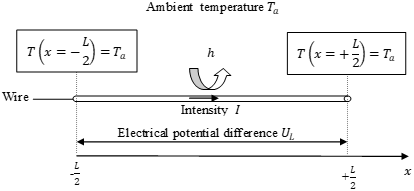Figure 1. Schema of the method used for thermal conductivity measurement of an electric conducting wire

Most of the methods used for the estimation of the thermal conductivity of electric conducting wires are based on the typical experimental device represented in Figure 1: The two extremities of a wire of length L are kept at a constant temperature Ta  and they are connected to an electrical generator. An electric current l gets through the wire, producing by Joule effect.

The mean temperature rise in the wire is deduced from the variation of its electrical resistance R measured by a four probe method. It is assumed that the wire electrical resistance varies linearly with its mean temperature as follows:

$R={{R}_{0}}[1+\alpha (\overline{T}-{{T}_{a}})]$ (1)

where $\overline{T}$ is the wire mean temperature defined as:

$\bar{T}\text{=}\frac{1}{L}\int _{0}^{L}T(x)dx$ (2)

α is the temperature coefficient of the electrical resistance and R0 is the electrical resistance of the wire at the temperature Ta. The heat produced inside the wire is transferred to its environment by two ways:

• Heat conduction from the wire to the extremities maintained at ambient temperature Ta.
• Combined convection-radiation heat transfer with a global coefficient  h to the surrounding environment. Thus the mean temperature rise $\overline{θ}$ depends on the following parameters:
• Time t (or pulsation ω in case of a periodic heat flux)
• Thermal conductivity λ (W m-1 K-1) and volumetric heat capacity ρc (J m-3 K-1) of the wire
• External (convection+radiation) heat transfer coefficient h (W m-2 K-1)
• Dimensions of the wire: diameter D and length L

The methods based on the previously described device may be classified in two types:

• Steady-state : in this case the electrical current intensity in the wire is constant: I=I0 and the steady state value $\overline{θ}$ of the mean temperature rise only depends on the wire thermal conductivity λ and on the external heat transfer coefficient h, Thus, λ can be deduced from a the steady-state measurement only if h is known. Most often the experiment is carried out under vacuum and h is considered to be null.
• Periodic  to : in this case I=I0  cos(ωt) and the measurement is repeated for several values of the electrical current pulsation w. The third harmonic U of electric potential difference UL is measured when the steady-state periodic regime is reached. A theoretical relation U=f(ω,λ,ρc,h) may be established from heat transfer modeling in the wire. The thermal conductivity λ may then be estimated from measurements corresponding to different values of ω if the sensitivities of U to λ, ρc and h are not correlated.

The proposed method intends to conserve the simplicity of the stationary method but without being forced to neglect the external heat transfer. This is made possible by realizing several steady-state measurements for different lengths of the same wire. The idea has already been used by Volkein and Kessler  for thin films thermal conductivity measurements but the applicability was not fully demonstrated. This paper will to demonstrate that thermal conductivity of wires may be estimated with a good precision using the proposed method.

The remainder of this paper is organized as follows: Section 2 describes the experimental device and the measurement method. Section 3 presents the modeling of the wire mean temperature rise θ by heat transfer analysis, both with a sensitivity study of this temperature rise θ to the length L of the wire, to its thermal conductivity λ and to the heat transfer coefficient h. Section 4 presents the experimental results obtained with Copper and Chromel wires, confirming the feasibility of thermal conductivity estimation by the proposed method.

2. Materials and Method

The experimental device is represented in Figure 2.

## f2.png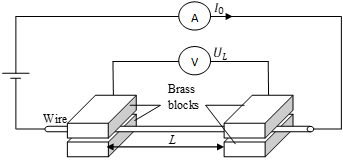Figure 2. Schema of the experimental device

Both extremities of the wire that must be characterized are sandwiched and pressed between two brass blocks: their high thermal conductivity ensures a uniform temperature in the blocks and their high thermal capacity ensures a constant temperature (equal to the environment temperature Ta ) during the experiment. The distance between the two brass blocks is measured by a caliper with a 0.02 mm precision.  The wire extremities are connected to a Tektronik PWS2185 Power Supply that produces (and measures) a constant intensity current Ithrough the wire. The electric potential difference between the two brass blocks (adding no electric resistance since the electrical conductivity of brass is high) is measured by a Tektronik DMM4040 Multimeter. To reduce the convective part in the external heat transfer, the device is set in a vacuum chamber where the pressure may be lowered to 3´10-2 mbar.

The wire means temperature rise $\bar{θ}=\bar{T}-T_a$  is related to the stationary value $U_L$  of the electrical potential difference by Eq. (1). Thus, the measurement of $U_L$  and of $I_0$  enable to estimate the wire mean temperature rise by:

$\bar{θ}=\bar{T}-T_a=\frac{1}{α}(\frac{U_L}{R_0I_0}-1)$ (3)

where:

• R0  is the electrical resistance of the wire at the ambient temperature Ta . The value of R0  is measured just before heating by a four probes method using a Tektronik DMM4040 Multimeter.
• α  is the temperature coefficient of the wire resistance obtained by the following measurement process: for each studied material, the electric resistance of a wire with a length has been measured as a function of the temperature by the multimeter. The wire was set in a temperature controlled enclosure Binder KB115 which temperature has been varied from 20°C to 60°C with a step of 10°C. During this process, the current intensity crossing the wire for the measurement of its electrical resistance is very low and does not produce a significant temperature rise. Two type K thermocouples were set on the junctions of the wire with the probe to verify that the wire temperature is identical to the enclosure one. Considering Eq. (1), the temperature coefficient α is deduced from the slope k of the curve R=f($\bar{T}-T_a$) by:

α=$\frac{k}{R_{20°C}}$ (4)

The expression of $\bar{T}$  as a function of λ, h and L will now be developed and it will be shown that the measurement of UL  for several values of the wire length L  enables the estimation of the thermal conductivity λ of the wire.

3. Model

The following hypotheses have been assessed:

• The temperatures of the brass blocks are constant and equal to the surrounding temperature Ta.
• The radial thermal gradient inside the wire is negligible. Considering the following limit values of the parameters: h<10 W m-2 K-1, D<1 mm and λ>1 W m-1 K-1, the Biot number ${{B}_{i}}=\frac{hD}{\lambda}$ is lower than 0.01. This value justifies the hypothesis that the temperature radial gradient is negligible inside the wire.

## 3.png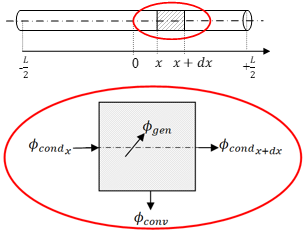Figure 3. Schema of the thermal balance on an infinitesimal part dx of the wire

For modeling the temperature T(x) of the wire, where x is the distance from the middle of the wire, a thermal balance has been realized on the wire part between x and x+dx  as represented on figure 3, it may be written:

${{\phi }_{con{{d}_{x}}}}+{{\phi }_{gen}}={{\phi }_{con{{d}_{x}}}}+{{\phi }_{conv}}$ (5)

${{\phi }_{con{{d}_{x}}}}\text{=-}\lambda \text{S(}\frac{dT}{dx}\text{)}$ (6)

${{\phi }_{gen}}={{\phi }_{0}}Sdx$ (7)

${{\phi }_{con{{d}_{x}}}}\text{=-}\lambda \text{S(}\frac{dT}{dx}{{\text{)}}_{x+dx}}$ (8)

${{\phi }_{conv}}=hPdx[T(x)-{{T}_{a}}]$ (9)

and: P is the wire perimeter: P = πD (D is the wire diameter)

S is the wire cross section: $S\text{=}\frac{\pi {{D}^{2}}}{4}$

ϕ0(x) is the heat flux per volume unit produced by Joule effect in the wire, given by:

${{\phi }_{0}}(x)=\frac{16{{\rho }_{0}}I_{0}^{2}}{{{\pi }^{2}}{{D}^{4}}}\{1+\alpha [T(x)-{{T}_{a}}]\}(W{{m}^{-3}})$ (10)

where ρ0 is the electrical resistivity of the wire (Ω m).

Setting θ(x)=T(x)-Ta, this system of equations leads to:

$\frac{{{d}^{2}}\theta }{d{{x}^{2}}}-\frac{hP}{\lambda S}\theta +\frac{{{\phi }_{0}}}{\lambda }=0$ 0≤x≤L/2  (11)

The boundary conditions are:

$\frac{d\theta }{dx}=0$ (null flux for symmetry reason) at x=0 (12)

θ = 0 at  x=L/2 (13)

Eq. (11) can also be written:

$\frac{{{d}^{2}}\theta }{d{{x}^{2}}}-(\frac{4h}{\lambda D}-\frac{16{{\rho }_{0}}\alpha I_{0}^{2}}{\lambda {{\pi }^{2}}{{D}^{4}}})\theta +\frac{16{{\rho }_{0}}I_{0}^{2}}{\lambda {{\pi }^{2}}{{D}^{4}}}=0$ (14)

If: $\frac{4h}{\lambda D}-\frac{16{{\rho }_{0}}\alpha I_{0}^{2}}{\lambda {{\pi }^{2}}{{D}^{4}}}>0$, let us consider:

$K=\frac{16{{\rho }_{0}}I_{0}^{2}}{\lambda {{\pi }^{2}}{{D}^{4}}}$ (15)

And: $\beta _{1}^{2}=\frac{1}{\lambda }(\frac{4h}{D}-\frac{16\alpha {{\rho }_{0}}I_{0}^{2}}{{{\pi }^{2}}{{D}^{4}}})$ (16)

Taking into account the boundary conditions Eq. (12) and Eq. (13) the resolution of Eq. (14) leads to:

$\theta (x)=\frac{k}{\beta _{1}^{2}}[1-\frac{\cosh ({{\beta }_{1}}x)}{\cosh ({{\beta }_{1}}L/2)}$ (17)

The mean temperature rise can be calculated by:

$\bar{\theta}(x)=\frac{2}{L}\int_0^{L/2}\theta (x)dx$ (18)

And finally:

$\overline{\theta }=\overline{T}-{{T}_{a}}=\frac{K}{\beta _{1}^{2}}[1-\frac{2\tanh ({{\beta }_{1}}L/2)}{{{\beta }_{1}}L}]$ (19)

If $\frac{4h}{\lambda D}-\frac{16{{\rho }_{0}}\alpha I_{0}^{2}}{\lambda {{\pi }^{2}}{{D}^{4}}}<0\\let us consider: \,\,\,\,\beta _{2}^{2}=\frac{1}{\lambda }(\frac{16{{\rho }_{0}}I_{0}^{2}}{{{\pi }^{2}}{{D}^{4}}}-\frac{4h}{\lambda D})$ (20)

Taking into account the boundary conditions Eq. (12) and Eq. (13) the resolution of Eq. (14) leads to:

$\theta (x)=\frac{k}{\beta _{2}^{2}}[\frac{\cos ({{\beta }_{2}}x)}{\cos ({{\beta }_{2}}L/2)}-1]$ (21)

Eq. (18) and Eq. (21) lead to:

$\bar{\theta }=\bar{T}-{{T}_{a}}=\frac{K}{\beta _{2}^{2}}[1-\frac{2\tan ({{\beta }_{2}}L/2)}{{{\beta }_{2}}L}-1]$ (22)

With the hypotheses that h=0 and that the heat flux ϕ0 dissipated in the wire by Joule effect is constant and equal to:

${{\phi }_{0}}=\frac{4UI_{0}^{2}}{\pi {{D}^{2}}L}$ (23)

Eq. (14) becomes:

$\frac{{{d}^{2}}\theta }{d{{x}^{2}}}+\frac{{{\phi }_{0}}}{\lambda }=0$ (24)

Let us consider: ${{K}_{1}}=\frac{{{\phi }_{0}}}{\lambda }$  (25)

The resolution of Eq. (24) taking into account the boundary conditions Eq. (12) and Eq. (13) lead to:

$\theta (x)={{K}_{1}}(\frac{{{L}^{2}}}{4}-\frac{{{x}^{2}}}{2})$ (26)

And finally: $\bar{\theta }=\bar{T}-{{T}_{a}}=\frac{1}{3}\frac{U{{I}_{0}}L}{\lambda \pi {{D}^{2}}}$ (27)

Then the thermal conductivity λ of the wire can be deduced by:

$\lambda =\frac{1}{3}\frac{U{{I}_{0}}L}{\pi {{D}^{2}}\overline{\theta }}$ (28)

Several authors such as Moon  estimate the thermal conductivity l by using Eq. (28). It will be further shown than in some cases using Eq. (28) may lead to an estimation error up to several percents so that it is always better to use Eq. (19) or Eq. (22).

4. Sensitivity Analysis

The reduced sensitivities of the temperature $\overline{T}(L)$ to the parameters λ and h are defined as $\lambda \frac{\partial \overline{T}}{\partial \lambda }(L)$ and $h\frac{\partial \overline{T}}{\partial \lambda }(L)$. If they are not proportional on a wire length interval [L1; L2] then it will be possible to estimate separately λ and h from several mean temperature measurements realized for different wire lengths in this interval .

The values of $\lambda \frac{\partial \overline{T}}{\partial \lambda }(L)$ and $h\frac{\partial \overline{T}}{\partial \lambda }(L)$have been calculated numerically by Eq. (19) or Eq. (22), for example:

$\lambda \frac{\partial \overline{T}}{\partial \lambda }(L)=\lambda \frac{\overline{T}(1.001\lambda ,h,L)}{0.001\lambda }$ (29)

The three following practical cases (corresponding to further experiments) have been analyzed:

• A Chromel wire with a diameter D=0.254 mm
• A Chromel wire with a diameter D=0.127 mm
• A Copper wire with a diameter D=0.0254 mm.

The following thermal properties have been considered in the simulations:

• Copper: λ=386 W m-1 K-1, ρ_0=1.4×10-8 Ω m, α=4.0×10-3 K-1.
• Chromel: λ=17.4 W m-1 K-1, ρ0=7.06×10-7 Ω m, α=3.3×10-3 K-1.

The calculations have been done with an external heat transfer coefficient h=2 W m-2 K-1 corresponding to an experiment under vacuum where convection may be neglected and with a wire emissivity around 0.3.

Figures 4 to 6 represent for each material the following parameters as a function of the wire length L:

• The mean temperature rise $\overline{\theta }$ calculated on one hand by Eq. (19) or Eq. (22) and on the other hand by Eq. (28).
• The reduced sensitivities of the mean temperature $\overline{T}$ to the thermal conductivity λ and to the external heat transfer coefficient h.
• The ratio of these two reduced sensitivities.

Figures 4 to 6 show that the precision of Eq. (28) (in which the external heat transfer is neglected) decreases when the wire length or its diameter increases. One can also see on figure 6 that for a Copper wire with a length lower than 2 cm the sensitivity of the mean temperature to the thermal conductivity λ is at least thirty times greater than its sensitivity to the external heat transfer coefficient h. It is thus possible to fix h to an approximate value and then to deduce l solving Eq. (19) or Eq. (22) from a single temperature measurement realized with a wire length lower than 2 cm.

Concerning the thinner Chromel wire, the length should be lower than 1 cm to obtain a ratio of the sensitivities to λ and to h to greater than 30. The uncertainty measurement on a wire with a length lower than 1 cm may become significant so that in this case it is better to realize several measurements with different wire lengths Li to estimate separately the thermal conductivity λ and the external heat transfer h. This separate estimation is possible because the ratio of the sensitivities represented in Figure 4 and 5 is not constant when the wire length varies. The estimation will be realized by minimizing the sum of the quadratic difference defined as:

$S\text{=}\sum\limits_{i=1}^{n}{{{[{{\overline{T}}_{\exp }}({{L}_{i}})-{{\overline{T}}_{\bmod }}({{L}_{i}})]}^{2}}}$ (30)

where n is the number of wire lengths Li tested, using the Levenberg-Marquart algorithm .

## 4.png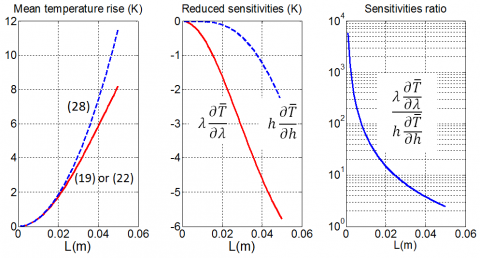Figure 4. Mean temperature rise and reduced sensitivities of $\overline{T}$ for a Chromel wire with a diameter D=0.254 , (I0=0.06 A)

## 5.png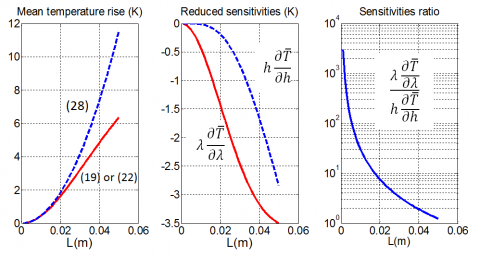Figure 5. Mean temperature rise and reduced sensitivities of $\overline{T}$ for a Chromel wire with a diameter D=0.127 mm (I0=0.015)

## 6.pngFigure 6. Mean temperature rise and reduced sensitivities of $\overline{T}$ for a Copper wire with a diameter D=0.0254 mm (I0=0.02 A)

5. Experimental Results

First, the temperature coefficients for the electrical resistance of each wire were measured. The experimental results are: αCopper=3.88×10-3 K-1 and αChromel=3.26×10-3 K-1. The first value is very close to the value αCopper=3.93×10-3 K-1 given by Fallou .

Eq. (15) shows that K is proportional to D4. Thus, the relative standard deviation of K would be four times the standard deviation of D if Eq. (15) was used to calculate K. Since the measurement of the electrical resistance of a thin wire is much more accurate than the measurement of its diameter, the resistance per length unit $\frac{R}{L}$ of each wire has been measured and then K has been calculated by:

$K\text{=}\frac{16(1+\alpha \theta )I_{0}^{2}}{\lambda \pi {{D}^{2}}}\frac{R}{L}$ (31)

Moreover, when using Eq. (31) instead of Eq. (15), it is no longer necessary to know the value of the electrical resistivity ρ0 of the wire to calculate K.

Table 1 presents the measured values for each of the three wires.

The mean temperature $\overline{T}$ of a Copper wire with a length L=2.1 cm and a diameter D=0.025 mm has been measured using the experimental device described in Figure 1, under an air pressure of 3×10-2 mbar. The measurement has been repeated three times.

Table 1. Experimental values of the linear resistance of the wires

 Chromel Chromel Copper D[mm] 0.127 0.254 0.025 L [m] 1.044 1.070 0.200 R [Ω] 55.81 15.33 6.987 $\frac{R}{L}$ [Ω m-1] 53.46 14.33 34.94

The measured values of $\overline{T}$ and the values of the thermal conductivity λ calculated by Eq. (19) or Eq. (22) on one hand and by Eq. (28) on the other hand are presented in Table 2. For the estimation of using Eq. (19) or Eq. (22), two limit values hmin and hmax of the external heat transfer coefficient h have been considered, enabling the estimation of two extreme values λmin and λmax of λ.

Table 2. Experimental values of $\overline{T}-T_a$; estimated and reference value of the thermal conductivity λ of Copper

 D  [m] 0.025 mm $\overline{T}-T_a$ [K] 16.65 K $s\overline{T}$ [K] 0.12 K λ [W m-1 K-1] Eq. (19) (h=6 W m-2 K-1) 393.0 Eq. (19) (h=2 W m-2 K-1) 379.6 Eq. (28) 370.9 Reference  386

The low pressure value decreases the convective heat transfer and the external heat transfer is essentially done by radiation. The radiation heat transfer coefficient of a surface at temperature T is lower than 4σT3, where σ is the Stefan-Boltzmann constant (σ=5.67×10-8 W m-2 K-4), corresponding to an approximate value of 6 W m-2 K-1 for a black body at a temperature of 25°C. Thus, the extreme values hmin=2 W m-2 K-1 and hmax=6 W m-2 K-1 have been considered.

Compared to a reference value of  386 W m-1 K-1 , the relative deviation is less than 2 % that is quite acceptable. It may be noted that using the approximate Eq. (28) would lead to a greater deviation around 4%.

For the Chromel wires the mean temperatures of wires with lengths between 15 mm and 85 mm have been measured under a constant pressure of 6.10-2 mbar. An algorithm minimizing the sum of the quadratic errors between the experimental values and the values calculated with Eq. (19) enabled the simultaneous estimation of the thermal conductivity λ and of the external heat transfer coefficient h for each wire. The experimental points and the theoretical curves are presented in Figure 7. The values of λ  and h obtained using Eq. (19) are presented in Table 3.

Furthermore, the specific heat c of the Chromel has been measured with a Setaram mdsc3 differential scanning calorimeter and its density ρ has been estimated by weighing a sample and measuring its dimensions. The thermal diffusivity a has been estimated by the flash method  applied on a plane sample.  The thermal properties obtained at 20°C are:

ρc=3.83×106 J m-3 K-1, a=4.54×10-6 m2 s-1

leading to λ=17.4 W m-1 K-1.

The estimated values of the Chromel thermal conductivity obtained by our method are very close to the values obtained by coupling the results of the calorimetric and flash methods, with a deviation less than 4% that is quite acceptable.

## 7a.png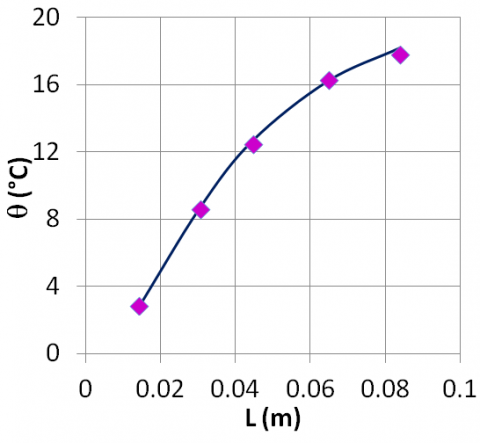## 7b.png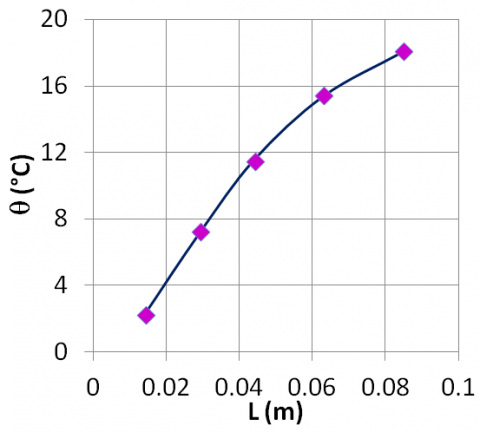Figure 7. Experimental (□) and modeled (  ) temperature for Chromel wires: a) D=0.127 mm and b) =0.254 mm

Table 3. Estimated values of  the wire thermal conductivitiy λ and of the external heat transfer coefficient h for two Chromel wires

 Chromel Wire λ h [W m-1 K-1] [W m-2 K-1] Experiment D=0.127 mm 18.0 5.8 D=0.254 mm 18.2 4.5 Reference - 17.4 -
6. Conclusions

The steady-state method proposed in this paper enables the measurement of the thermal conductivity l of an electrical conducting wire or fiber. The expression of the wire mean temperature has been established as a function of the wire thermal conductivity l and of the external heat transfer coefficient h. This expression has been used to realize a sensitivity analysis that demonstrated the possibility of the simultaneous estimation of l and h from several steady-state measurements carried on different wire lengths. The estimation of l  is realized without making the assumption that the external heat transfer is null. The theoretical model and the estimation method have been validated by an experimental study carried out with a Chromel wire and with a Copper wire. The estimated values of the thermal conductivities are in good agreement with references values (less than 5 % deviation).

The extension of the method to thinner and shorter wires or fibers

Nomenclature
 D wire diameter, m h external heat transfer coefficient, W.m-2.K-1 L wire length, m n number of wire lengths tested P wire perimeter, m R electric resistance, W R0 electric resistance at initial temperature, W S wire section area, m2 s$\bar{T}$ standard deviation of $\bar{T}$, °C T wire temperature, °C Ta ambient temperature, °C $\bar{T}$ wire mean temperature, °C x distance from the middle of the wire, m Greek symbols a temperature coefficient of the wire electrical resistance, K-1 ϕ0 heat flux per volume unit, W.m-3 λ thermal conductivity, W.m-1.K-1 ρ density, kg.m-3 ρ0 wire electrical resistivity, W.m θ wire temperature rise, K $\bar{θ}$ wire mean temperature rise, K ω alternative current pulsation, rd.s-1
References

 Bonura M, Senatore C. (2013). Thermal conductivity of industrial Nb3Sn wires fabricated by various techniques. IEEE Transactions on Applied Superconductivity 23(3): 6000404. https://doi.org/10.1109/TASC.2012.2234203

 Moon J, Weaver K, Feng B, Chae HG, Kumar S, Baek JB, Peterson GP. (2012). Thermal conductivity measurement of individual poly(ether ketone)/carbon nanotube fibers using a steady-state dc thermal bridge method. Review of Scientific Instruments 83: 016103. https://doi.org/10.1063/1.3676650

 Bougeois O, Fournier T, Chaussy J. (2007). Measurement of the thermal conductance of silicon nanowires at low temperature. Journal of Applied Physics 101: 016104. https://doi.org/10.1063/1.2400093

 Bhatta RP, Mohr RK, Brandys M, Pegg IL, Dutta B. (2010). High temperature thermal conductivity of platinium microwire by 3ω method. Review of Scientific Instruments 81: 114904. https://doi.org/10.1063/1.3496048

 Lu L, Yi W, Zhang DL. (2001). 3ω method for specific heat and thermal conductivity measurements. Review of Scientific Instruments 72: 2996. https://doi.org/10.1063/1.1378340

 Zhang X, Fujiwara S, Fujii M. (2000). Short-hot-wire method for the measurement of the thermal conductivity of a fine fibre. High Temperatures-High Pressures 32: 493-500. https://doi.org/10.1068/htwu374

 Choi TY, Poulikakos D, Tharian J, Sennhauser U. (2005). Measurement of thermal conductivity of individual multiwalled carbon nanotubes by the 3ω method. Applied Physics Letters 87: 013108. https://doi.org/10.1063/1.1957118

 Dames C, Chen G. (2000). 1ω, 2ω, 3ω methods for measurements of thermal properties. Review of Scientific Instruments 76: 124902. https://doi.org/10.1063/1.2130718

 Liang J, Saha MC, Altan MC. (2013). Measurement of thermal conductivity of carbon fibers using wire-based 3 omega method. 28th Technical Conference of the American-Society-for-Composites, State College, Pennsylvania University, USA, pp. 593-599.

 Volklein F, Kessler E. (1984). A method for the measurement of thermal conductivity, thermal diffusivity, and other transport coefficients of thin films. Physica Status Solidi 81(2): 585-596. https://doi.org/10.1002/pssa.2210810222

 Beck JV, Arnold KJ. (1977). Matrix Analysis for linear parameter estimation in parameter estimation in engineering and science. John Wiley and Sons, New York. https://doi.org/10.1002/aic.690240233

 Marquart DW. (1963). An algorithm for least-squares estimation of nonlinear inequalities. J. Appl. Math. 11: 431-441.

 Fallou M. (1998). Résistivité des métaux. Techniques de l’Ingénieur, Paris

 Holman JP. (1990). Heat transfer. 7th edition. McGraw-Hill, New York